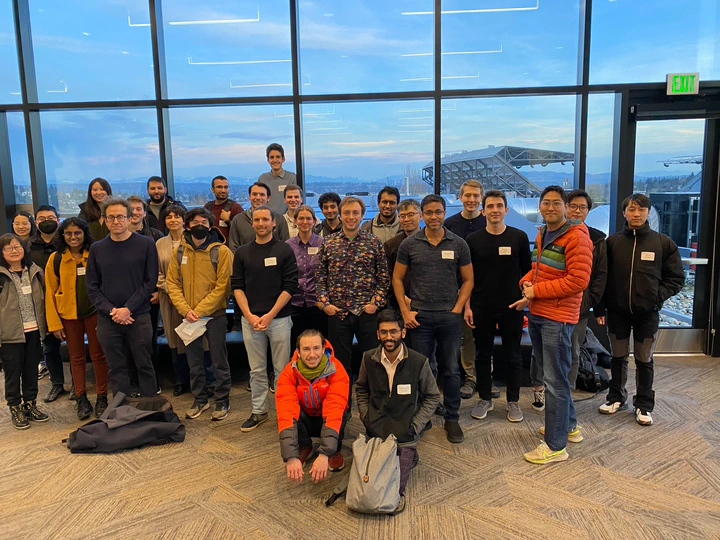# IFML+KI retreat 2023KI Retreat - group photo

Date
2023, Feb 2 9:30 AM PST — 6:10 PM PST
Event
IFML+KI retreat 2023
Location
Zillow Commons, 4th floor, Gates Center

• Soumik Pal
• Sewoong Oh

## Abstracts

Overparametrization in machine learning: insights from linear models
Andrea Montanari (Stanford)
Deep learning models are often trained in a regime that is forbidden by classical statistical learning theory. The model complexity can be larger than the sample size and the train error does not concentrate around the test error. In fact, the model complexity can be so large that the network interpolates noisy training data. Despite this, it behaves well on fresh test data, a phenomenon that has been dubbed `benign overfitting.' I will review recent progress towards a precise quantitative understanding of this phenomenon in linear models and kernel regression. In particular, I will present a recent characterization of ridge regression in Hilbert spaces which provides a unified understanding on several earlier results. [Based on joint work with Chen Cheng]
Towards Instance-Optimal Algorithms for Reinforcement Learning
Kevin Jamieson (UW + IFML)
The theory of reinforcement learning has focused on two fundamental problems: achieving low regret, and identifying epsilon-optimal policies. While in multi-armed bandits there exists a single algorithm that is instance-optimal for both, I will show in this talk that for tabular MDPs this is no longer possible—there exists a fundamental tradeoff between achieving low regret and identifying an epsilon-optimal policy at the instance-optimal rate. That is, popular algorithms that exploit optimism cannot be instance optimal. I will then present an algorithm that achieves the best known instance-dependent sample complexity for PAC tabular reinforcement learning which explicitly accounts for the sub-optimality gaps and attainable state visitation distributions in the underlying MDP. I will then discuss our recent work in the more general linear MDP setting where we have proposed an algorithm that is qualitatively very different but nevertheless achieves an instance-dependent sample complexity.
Towards a Mathematical Theory of Development
Geoff Schiebinger (UBC + KI)
This talk introduces a mathematical theory of developmental biology, based on optimal transport. While, in principle, organisms are made of molecules whose motions are described by the Schödinger equation, there are simply too many molecules for this to be useful. Optimal transport (OT) provides a set of equations that describe development at the level of cells. We leverage OT to analyze single-cell RNA-sequencing datasets and shed light on questions like: How does a stem cell transform into a muscle cell, a skin cell, or a neuron? How can we reprogram a skin cell into a stem cell?
Git Re Basin Merging Models modulo Permutation Symmetries
Jon Hayase (UW + IFML)
The success of deep learning is due in large part to our ability to solve certain massive non-convex optimization problems with relative ease. Though non-convex optimization is NP-hard, simple algorithms – often variants of stochastic gradient descent – exhibit surprising effectiveness in fitting large neural networks in practice. We argue that neural network loss landscapes contain (nearly) a single basin after accounting for all possible permutation symmetries of hidden units a la Entezari et al. (2021). We introduce three algorithms to permute the units of one model to bring them into alignment with a reference model in order to merge the two models in weight space. This transformation produces a functionally equivalent set of weights that lie in an approximately convex basin near the reference model. Experimentally, we demonstrate the single basin phenomenon across a variety of model architectures and datasets, including the first (to our knowledge) demonstration of zero-barrier linear mode connectivity between independently trained ResNet models on CIFAR-10 and CIFAR-100. Additionally, we investigate intriguing phenomena relating model width and training time to mode connectivity. Finally, we discuss shortcomings of the linear mode connectivity hypothesis, including a counterexample to the single basin theory.
Scaling limit of SGD over large networks
Raghav Tripathi (UW+IFML+KI)
Wasserstein gradient flows often arise from mean-field interactions of exchangeable particles. In many interesting applications however, the “particles” are edge weights in a graph whose vertex labels are exchangeable but not the edges themselves. We investigate the optimization of functions over this class of symmetries. Popular applications include training of large computational graphs like (Deep) Neural Networks. We show that discrete noisy stochastic optimization algorithms over finite graphs have a well-defined analytical scaling limit as the size of the network grows to infinity. The limiting space is that of graphons, a notion introduced by Lovász and Szegedy to describe limits of dense graph sequences. The limiting curves are given by a novel notion of McKean-Vlasov equation on graphons and a propagation of chaos phenomenon can be observed to hold. In the asymptotically zero-noise case, the limit is a gradient flow on the metric space of graphons.
Developmental trajectory inference in the presence of a growth-induced bias in clonal data
Becca Bonham-Carder (UBC (now at Mission Control Space Services) + KI)
Developmental trajectory inference is the task of estimating the paths followed by cells over time as they develop (divide, die and differentiate) in a biological population. In this work we consider the problem of inferring developmental trajectories at single-cell resolution from time courses of dynamic populations which contain observations of cell developmental state and shared ancestry through lineage tracing with DNA barcodes. A group of cells sharing a common barcode/ancestor are referred to as a clone. We identify and explore a statistical phenomenon that may emerge in this inference setting, namely how the relative growth rates of cells influence the probability that they will be sampled in clones observed across multiple time points. We consider how this sampling bias affects state-of-the-art methods for this inference problem, including optimal transport approaches, and how one might design methods that are robust to this bias.